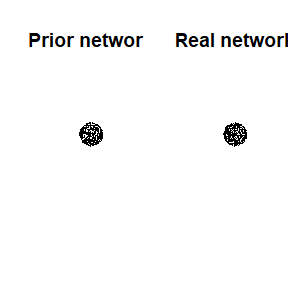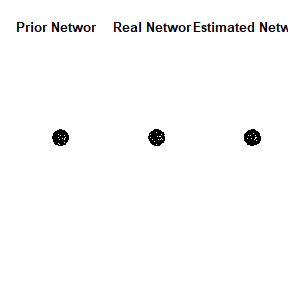Overview of using SBIC for network models

library(SBICgraph)
#>
#> Attaching package: 'SBICgraph'
#> The following object is masked from 'package:stats':
#>
#>     simulate
library(network) # for visualization
#> network: Classes for Relational Data
#> Version 1.16.1 created on 2020-10-06.
#> copyright (c) 2005, Carter T. Butts, University of California-Irvine
#>                     Mark S. Handcock, University of California -- Los Angeles
#>                     David R. Hunter, Penn State University
#>                     Martina Morris, University of Washington
#>                     Skye Bender-deMoll, University of Washington
#>  For citation information, type citation("network").
#>  Type help("network-package") to get started.

# to reset par
resetPar <- function() {
dev.new()
dev.off()
op
}

The function comparison allows for comparison between the true network and the estimated network from the SBIC method.

First we create a simulated data set using the embedded simulate function within SBIC. The function simulate generates a data frame, a real network adjacency matrix and a prior network adjacency matrix.

p <- 200
m1 <- 100
m2 <- 30
d <- simulate(n=100, p=p, m1=m1, m2=m2)
data<- d$data real<- d$realnetwork
priori<- d$priornetwork We can visualize the networks prior_net <- network(priori) real_net <- network(real) par(mfrow = c(1,2)) plot(prior_net, main = "Prior network") plot(real_net, main = "Real network")par(resetPar()) We examine some features of both the prior network and the real network sum(priori[lower.tri(priori)]) #>  100 sum(priori[lower.tri(priori)])/(p*(p-1)/2) #>  0.005025126 sum(real[lower.tri(real)]) #>  100 sum(real[lower.tri(real)])/(p*(p-1)/2) #>  0.005025126 Then we can fit SBIC using one function lambda<- exp(seq(-10,10, length=30)) # calculating the error rate from the number of edges in the true graph and the number of discordant pairs r1 <- m2/m1 r2 <-m2/(p*(p-1)/2-m1) r <- (r1+r2)/2 model<- sggm(data = data, lambda = lambda, M=priori, prob = r) Comparing the estimated network to the true and prior network. Our comparison function above calcualtes the Positive selection rate (PSR) and the False positive rate (FDR) print("Comparing estimated model with the real network") #>  "Comparing estimated model with the real network" comparison(real = real, estimate = model$networkhat)
#> $PSR #>  0.4 #> #>$FDR
#>  0.4666667
print("Comparing the prior network with the real network")
#>  "Comparing the prior network with the real network"
comparison(real = real, estimate = priori)
#> $PSR #>  0.7 #> #>$FDR
#>  0.3

We can also compare visualizations

estimated_net <- network(model$networkhat) par(mfrow = c(1,3)) plot(prior_net, main = "Prior Network") plot(real_net, main = "Real Network") plot(estimated_net, main = "Estimated Network")par(resetPar()) The model object also stores all the candidate models generated. length(model$candidate)
#>  64